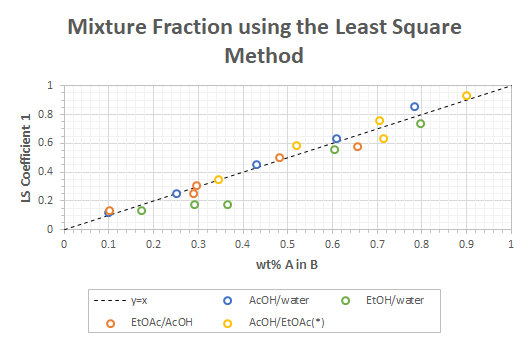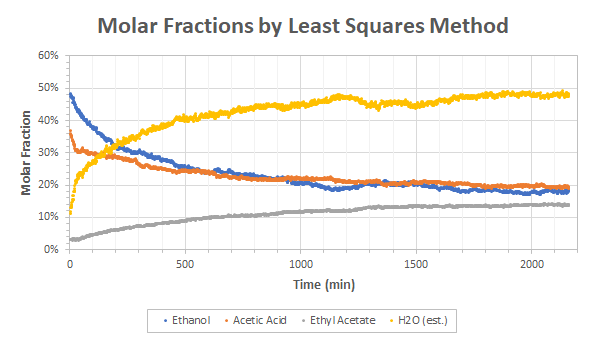# Proportionality of the Least Square Coefficients in Raman Spectroscopy

I recently shared some results about measuring the kinetics of the ethyl acetate reaction. At the time, I was not entirely satisfied by the results due to the fact that I didn’t know what was the exact relation between the returned coefficients of the least square method and the actual fraction content of each chemicals in the mixture. I assumed that the returned coefficients were molar fractions and we will see here that was unfortunately not correct.

Even before that, I rana test to see how much methanol I could detect in ethanol. The results correlated well to weight fractions but it was difficult to judge due to two factors: (1) ethanol and methanol have relatively close molar masses and, (2) I only went up to 30% of methanol. Nonetheless, I obtained a very satisfactory 0.6% probable error of methanol in ethanol using that technique with a part of the error due to the laboratory scale I used.

It was therefore logical to study how mixtures of the components involved in the esterification reaction would correlate to the least square coefficients by looking at the weight fraction first. The results are presented in Figure 1. Note that the data of AcOH/EtOAc were taken from the same dilution experiment as the data of EtOAc/AcOH (it is therefore the second coefficient returned by the least square method).Figure 1 – Correlation between the weight fraction and least square coefficients. (*) From the same experiment as EtOAc/AcOH.

Apart from two points in the ethanol/water dilution, all the points line up relatively well to a direct y=x relationship with a probable error of 3.7% when including all points or 3.5% when excluding the two points of ethanol/water that are a bit off near 30-40%.

Again, a part of the error is due to the laboratory scale I used to perform the dilution. I experienced some weird drift during the dilutions as everytime I emptied the vials to refill them, the zero shifted by -20 mg (so the emptied vial was each time 20 mg lighter than in the previous run – which make absolutely no sense). I don’t know what was causing the effect but I would not be surprised that about 1% of the error obtained was due to that factor.

Also, this time the molecules are very different in terms of scales/weights so there is no reason that they should follow a strict linear trend. My understanding of the phenomenon is that the least square coefficients are proportional to the probability of the laser hitting a specific molecule which would therefore correlates to the volume fraction of each component. However, as we study simple organic molecules, the volume occupied by each molecule is more or less correlated to the molar masses and hence the least square coefficients appear to be correlated to the weight fraction of the components. I would not be surprised if the correlation fails with strange molecules with more heteroatoms than carbon atoms or with heavy atoms like brome. I unfortunately don’t have such chemicals available at the moment for testing.

Nonetheless, translating the least square coefficients to weight fractions seems to be a reasonable approximation unless a detailed calibration is performed.

I therefore decided to re-run the esterification analysis using a conversion from the assumed returned weight fraction to a molar fraction. I obtained the results of Figure 2.Figure 2 – Ethyl Acetate reaction re-analysed using weight to molar fraction conversion

The results were unfortunately not as stunning as I would have expected. The equilibrium constant became a bit better with Keq=2 instead of 3.9 (previous value was 1 so it is already better). Also, this time the production of ethyl acetate matched the consumption of acetic acid and ethanol. Slightly more ethanol was consumed in the reaction (especially at the beginning) but that can probably be explained by partial evaporation of the ethanol as it is the most volatile compound in the mixture and that the reaction lasted for 36 hours. However, the production of water is completely off which makes the kinetic analysis (and to some extend the equilibrium constant) go crazy.

So, the story is not complete yet but we already progressed in the good direction! I will however move on and study new topics in the following months. I have already performed another experiment that I would like to present on this website and I have two other interesting experiments planned that I still have to run. Also, I would like to refocus on the spectrometer itself to progress towards a first functional prototype by the end of the year.

I would like to give a big thanks to James who has supported this post through Patreon. If you’d like to support me too and get credits as well as many other stuff, consider donating 🙂 You can make the difference!

Categories: Applications